Next: Continuous Up: A Bestiary of ... Previous: A Bestiary of ...

# Discrete

Binomial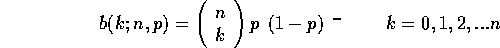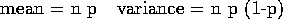Geometric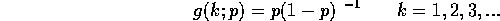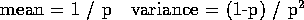A specialization of the Negative Binomial distribution, where the experiment is stopped after the first success.

Hypergeometric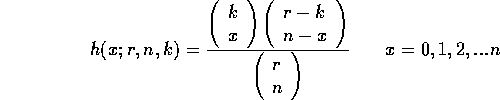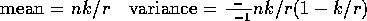Negative Binomial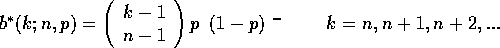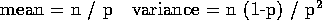Applies to problems where an experiment is done until a fixed number of successes ( n ) in a certain number of trials ( k ) occur. These types of experiments are called negative binomial experiments. The negative binomial random variable is k.

Poisson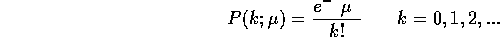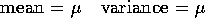Applies to processes with small constant probabilities. Is a good approximation to a binomial distribution for large n and small p.

Uniform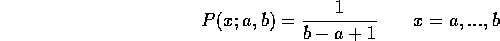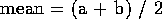Skip Carter
Wed May 22 15:29:40 PDT 1996